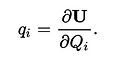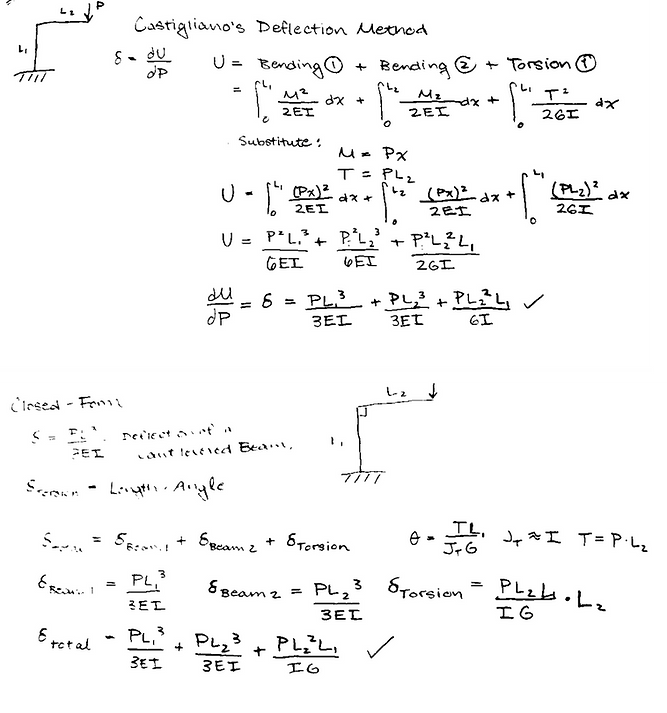###### Castigliano's Method | Seek n' GeekV

Castigliano's Method is a useful "method for determining the displacements of a linear-elastic system based on the partial derivatives of the energy."  His two theorems relate the change in energy with a force causing the change with the resulting displacement. Castigliano's Method is particularly useful for complex beam geometries that would be difficult to calculate otherwise.

Theorem 1: Forces in Elastic Structures 

If the strain energy of an elastic structure can be expressed as a function of generalized displacement qi then the partial derivate of the strain energy with respect to generalized displacement gives the generalized force Qi. In equation form: (U is strain energy)

Theorem 2: Displacements in Linearly-Elastic Structures 

If the strain energy of a linearly elastic structure can be expressed as a function of generalized force Qi, then the partial derivative of the strain energy with respect to generalized force gives the generalized displacement qi in the direction of Qi. In equation form:The classic proof of Castigliano's method is to solve a cantilevered beam problem with a force on the tip using Castigliano and compare to the standard equation. The example from the Wikipedia article uses Theorem 2 solve for the deflection of a beam. In the calculation U is the strain energy, P is the applied force, E is the modulus of elasticity and I is the moment of inertia of the beam.Solving for deflection, it is shown that the final simplified answer does indeed match the standard beam deflection equation.

I decided to solve another example with slightly more complexity to validate this simple proof.  As drawn below I calculated the deflection of a cantilevered right angle beam with a force P acting on the tip using both Castigliano's Method and a closed form. For the strain energy in Castigliano's method I summed the strain energies from bending and torsion, and substituted in values for M, moment, and T, torque.

In the closed form I summed the deflections resulting from the bending of beam 1, the bending of beam 2, and the angular offset due to torsion of beam 1 from the moment created by beam 2. As shown in the calculations below, the deflections calculated by the two methods are equal.Calculations of deflection using Castigliano's Method and the closed form